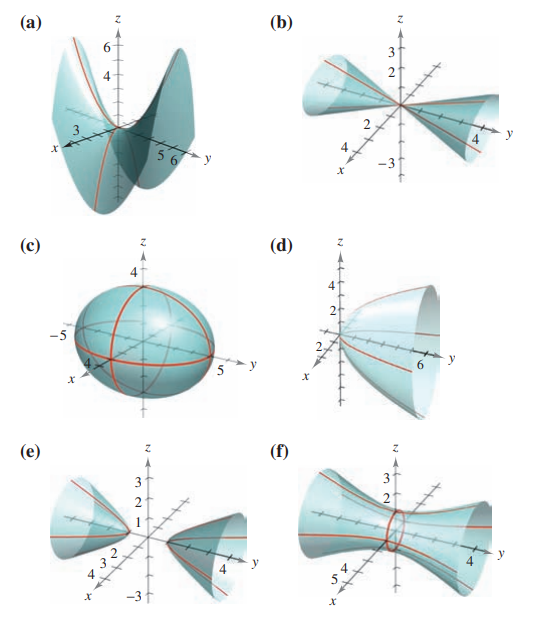×
Get Full Access to Calculus: Early Transcendental Functions - 6 Edition - Chapter 11.6 - Problem 2
Get Full Access to Calculus: Early Transcendental Functions - 6 Edition - Chapter 11.6 - Problem 2

×

# ?In Exercises 1–6, match the equation with its graph. [The graphs are labeled (a), (b), (c), (d), (e), and (f).]ISBN: 9781285774770 141

## Solution for problem 2 Chapter 11.6

Calculus: Early Transcendental Functions | 6th Edition

• Textbook Solutions
• 2901 Step-by-step solutions solved by professors and subject experts
• Get 24/7 help from StudySoup virtual teaching assistantsCalculus: Early Transcendental Functions | 6th Edition

4 5 1 302 Reviews
11
0
Problem 2

In Exercises 1–6, match the equation with its graph. [The graphs are labeled (a), (b), (c), (d), (e), and (f).]$$15 x^{2}-4 y^{2}+15 z^{2}=-4$$

Text Transcription:

15 x^2-4 y^2+15 z^2=-4

Step-by-Step Solution:

Step 1 of 5) Figure 6.1 A cross-section S(x) of the solid S formed by intersecting S with a plane Px perpendicular to the x-axis through the point x in the interval 3a, b4.

Step 2 of 2

##### ISBN: 9781285774770

Calculus: Early Transcendental Functions was written by and is associated to the ISBN: 9781285774770. The full step-by-step solution to problem: 2 from chapter: 11.6 was answered by , our top Calculus solution expert on 11/14/17, 10:53PM. This textbook survival guide was created for the textbook: Calculus: Early Transcendental Functions, edition: 6. Since the solution to 2 from 11.6 chapter was answered, more than 256 students have viewed the full step-by-step answer. This full solution covers the following key subjects: . This expansive textbook survival guide covers 134 chapters, and 10738 solutions. The answer to “?In Exercises 1–6, match the equation with its graph. [The graphs are labeled (a), (b), (c), (d), (e), and (f).] $$15 x^{2}-4 y^{2}+15 z^{2}=-4$$Text Transcription:15 x^2-4 y^2+15 z^2=-4” is broken down into a number of easy to follow steps, and 28 words.

## Discover and learn what students are asking

Calculus: Early Transcendental Functions : The Cross Product of Two Vectors in Space
?In Exercises 1-6, find the cross product of the unit vectors and sketch your result. $$\mathbf{j} \times \mathbf{k}$$

Statistics: Informed Decisions Using Data : Data Collection
?In Problems 1–5, provide a definition using your own words. 5. Designed experiment

Unlock Textbook Solution